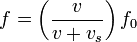# Doppler effect

## Homework Statement

A car approaches blowing its horn. The observed frequency is 503.7 Hz Assume that the speed of sound is 1100 ft/sec. the car's horn has a frequency of 450 Hz How fast is the car traveling.

?

## The Attempt at a Solution

I can't even figure out how to start. Sorry for my ignorance.

## Answers and Replies

berkeman
Mentor
Thread moved from Advanced to Intro Physics.

Try again to list the relevant equations. What equation relates the frequency, wavelength and velocity of sould waves? What equations are you shown in your text or other learning materials for the Doppler effect? What does the wikipedia.org page say about the Doppler effect?Is this it?

Thank You!